# High School Physics : Capacitors

## Example Questions

2 Next →

### Example Question #1 : Using Capacitor Equations

Three capacitors of equal capacitance are in a series circuit. If the total capacitance is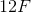, what is the capacitance of each individual capacitor?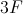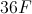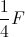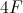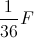Explanation:

The formula for capacitors in a series is: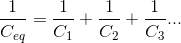Since we have three equal capacitors, we can say: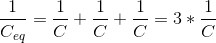Using the value for total capacitance, we can find the value for the individual capacitance.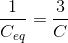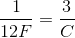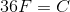### Example Question #2 : Using Capacitor Equations

Three capacitors of equal capacitance are in a parallel circuit. If the total capacitance is, what is the capacitance of each individual capacitor?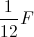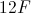Explanation:

The formula for capacitors in parallel is: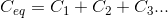Since we have three equal capacitors, we can say: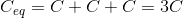Use the value for total capacitance to find the individual capacitance value.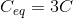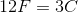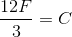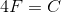2 Next →

### All High School Physics Resources